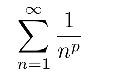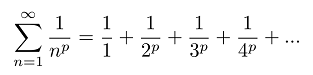# p-Series: Definition, Test for Convergence

Sequence and Series > p-series

A p-series takes the form:where p is a positive real number.

This notation represents an infinite sum, where n represents each natural number (whole, non-negative numbers that we use to count). In other words,## What is p-series Divergence?

Simply put, if a series diverges then it never settles (or sums to) a particular number. The harmonic series, where p=1, is divergent:But how can we know whether other types converge or diverge?

## The p-series test

A test exists to describe the convergence of all p-series. That test is called the p-series test, which states simply that:

• If p > 1, then the series converges,
• If p ≤ 1, then the series diverges.

Here are some examples of convergent series:Note the “p” value (the exponent to which n is raised) is greater than one, so we know by the test that these series will converge.

Here’s are some examples of divergent series. Note that the exponents are all less than one:The p-series test is a great test for quickly finding convergence for this special series type. Even the harmonic series follows the test; The series diverges for p = 1.

Just make sure that the series you’re trying to evaluate follows the general formula. Important to note though, is that a p-series is not the same as a geometric series; While a p-series has the index (n) in the denominator of the fraction, a geometric series will have the index (n) in the exponent of the base. Here they are, side by side:The series on the left is a p series, while the series on the right is a geometric series.

Also, the “p” is not short for power, as in power series, which has the basic form:## References

Benestad, C. et al. (2015). Power Series, Math 121 Calculus II. Retrieved January 3, 2020 from: https://mathcs.clarku.edu/~ma121/powerseries.pdf

Larson, R. & Edwards, B. (2013). Calculus. Cengage Learning; 10th edition

CITE THIS AS:
Stephanie Glen. "p-Series: Definition, Test for Convergence" From StatisticsHowTo.com: Elementary Statistics for the rest of us! https://www.statisticshowto.com/p-series-definition-test-for-convergence/